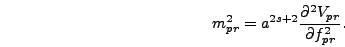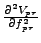Next: model_output(int flush, char *ext_) Up: Writing a Model File Previous: dvdf(int fld, INDEXLIST)

### effective_mass(float mass_sq[], float *field_values)

As explained in section 6.3.2 the effective mass used for initial conditions and for calculating spectra is given in program units by(4.17)

This function calculates the grid average offor each field and stores this square mass in the array mass_sq. Once again, you can look at the example file and easily see the equations from section 4.2.1. As with the potential energy function the effective mass function accepts a pointer to an array of field values. If this pointer is not NULL then these values should be used instead of the field values on the lattice.

In most of our model files the first part of the effective_mass() function consists of the calculation of the average squared values of the fields, either in terms of the lattice values or the array of field values passed to the function. Typically the derivativesdepend on these squared values. If this is the case for your model then you can leave this part of the function unchanged. The next part sets the square masses in terms of these values; these equations are the part of the function unique to each model. Finally the prefactoris added in. This last part should be the same for all models.Next: model_output(int flush, char *ext_) Up: Writing a Model File Previous: dvdf(int fld, INDEXLIST)